# Heptagon

A heptagon is a polygon that has seven sides (Hepta- means seven). In the figure below are several types of heptagons.

## Heptagon classifications

Like other polygons, a heptagon can be classified as regular or irregular. If all the sides and interior angles of a heptagon are equal, it is a regular heptagon. Otherwise it is an irregular heptagon.

Regular heptagonIrregular heptagon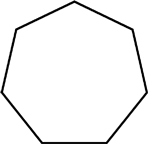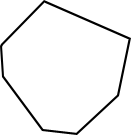All sides and interior angles are equal Not all sides and angles are equal

Heptagons and other polygons can also be classified as either convex or concave. If all interior angles of a heptagon are less than 180°, it is convex. If one or more interior angles is larger than 180°, it is concave. A regular heptagon is a convex heptagon. A concave heptagon is an irregular heptagon.

Convex heptagonConcave heptagon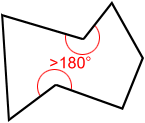All interior angles <180° One or more interior angles >180°

## Diagonals of heptagon

A diagonal is a line segment joining two non-consecutive vertices. A total of fourteen distinct diagonals can be drawn for a heptagon. The following figure is an example.

There are 4 diagonals extending from each of the 7 vertices of the heptagon above creating a total of 14 diagonals.

## Internal angles of a heptagon

The sum of the interior angles of a heptagon equals 900°.

As shown in the figure above, four diagonals can be drawn to divide the heptagon into five triangles. The blue lines above show just one way to divide the heptagon into triangles; there are others. The sum of interior angles of the five triangles equals the sum of interior angles of the heptagon. Since the sum of the interior angles of a triangle is 180°, the sum of the interior angles of the heptagon is 5 × 180° = 900°.

## Regular heptagon

A regular heptagon is a heptagon in which all sides have equal length and all interior angles have equal measure.

### Angles of a regular heptagon

Since each of the seven interior angles in a regular heptagon are equal in measure, each interior angle measures 900° ÷ 7 ≈ 128.57°, as shown below.

Each exterior angle of a regular heptagon has an equal measure that is approximately 51.43°.

### Symmetry in a regular heptagon

A regular heptagon has 7 lines of symmetry and a rotational symmetry of order 7, meaning that it can be rotated in such a way that it will look the same as the original shape 7 times in 360°.

Lines of symmetryRotational symmetry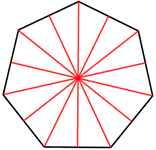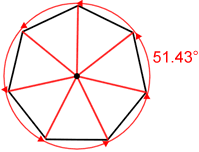7 lines of symmetry Seven 51.43-degree angles of rotation about the center